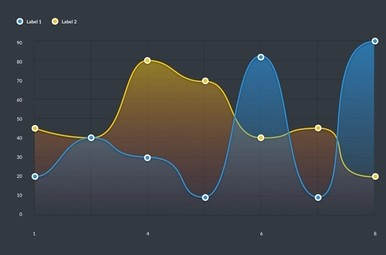# Chart - Line Intrpolation

Hello All,

is there any way how the figure.line can be interpolated by engine defaulty not to create addtionaly values by external function?

Thank you

JAn

@VASKOTechDesign I’m sorry but I don’t actually understand what you are asking. Bokeh line glyphs always draw all the points with linear segments in between. There are some options for controlling line joins, dashing, etc. but that is it. I don’t think that’s what you are referring to, though. It would probably help if you can point to something concrete (i.e. code) in another tool like MPL that is representative of what you are looking for.

Hello Bryan,

thank you for you super quick reply, I was about to prepare example, but you were quicker. So here is my simple example:

1. Bokeh Line Code:
``````import bokeh.plotting as bk
p = bk.figure()
x_axis_values = [1, 2, 3, 4, 5, 6, 7, 8, 9, 10, 11, 12, 13, 14, 15, 16,17,18]
y_axis_values = [4, 2, 8, 5, 6, 2, 10, 1, 3, 4, 5, 8 , 2, 16, 1, 2, 3, 4]
p.line(x_axis_values, y_axis_values)
p.circle(x=x_axis_values, y=y_axis_values, size=4, color="navy", alpha=0.5)
bk.show(p)
``````

here is result of the code:

1. Interpolated values by scipy code:
``````import bokeh.plotting as bk
import numpy as np
from scipy.interpolate import PchipInterpolator
p = bk.figure()
x_axis_values = [1, 2, 3, 4, 5, 6, 7, 8, 9, 10, 11, 12, 13, 14, 15, 16,17,18]
y_axis_values = [4, 2, 8, 5, 6, 2, 10, 1, 3, 4, 5, 8 , 2, 16, 1, 2, 3, 4]
Interpolate_range = len(x_axis_values) * 20
xvals=np.linspace(1, len(x_axis_values), Interpolate_range)
spl = PchipInterpolator(x = x_axis_values, y=y_axis_values, axis=1) # First generate spline function
y_smooth = spl(xvals) # then evalute for your interpolated points
p.line(xvals, y_smooth)
p.circle(x=x_axis_values, y=y_axis_values, size=4, color="navy", alpha=0.5)
bk.show(p)
``````

here is result of the code:

So the difference I wanted to describe is that you have to use addtional values to draw smooth line. But unfortuantelly it increase file size (if you use html). So my initial question is wheter there isinternal method of Line Figure to smooth it (to be more this style:)
→ not considerating the temlate of image

Thank you

@VASKOTechDesign Thanks for clarifying. There have been a few various past issues regarding “smooth paths”

but as of yet, nothing has been implemented. At present, Bokeh has built-in glyphs for `quadratic` and (cubic) `bezier` splines, but you would have to stitch together multiple splines manually, if you wanted one continuous curve of “higher” degree. For now, realistically, the main best option is simply to increase the discretization (i.e. ad more points).

Hello Bryan,

Okay thank you, I will use that SciPy interpolation to increase numvet of features.

You can close tihis issue.

Jan

This topic was automatically closed 90 days after the last reply. New replies are no longer allowed.